• 对称平均绝对百分比误差 (SMAPE)。 用法 smape（数据集） 计算数据集的对称平均绝对百分比误差。 此函数的范围是 (0, 200)。 例子 var smape = require ( 'smape' ) ; var dataset = [ { actual : 112.3 , ...JavaScript
• 对于回归预测结果，通常会有平均绝对误差、平均绝对百分比误差、均方误差等多个指标进行评价。这里，我们先介绍最常用的3个 目录 平均绝对误差（MAE） 均方误差（MSE）：均方根误差（RMSE）​ 平均绝对百分比...

对于回归预测结果，通常会有平均绝对误差、平均绝对百分比误差、均方误差等多个指标进行评价。这里，我们先介绍最常用的3个

目录

平均绝对误差（MAE）

平均绝对百分比误差 MAPE

平均绝对误差（MAE）

MAE 的值越小，说明预测模型拥有更好的精确度。The MAE is used to measure the average absolute error between the predicted value and the real value on the experimental data set. For a test set containing n microblog messages, MAE is defined as: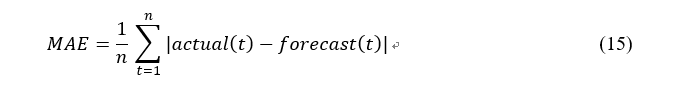import numpy as np

def mae_value(y_true, y_pred):
"""
参数:
y_true -- 测试集目标真实值
y_pred -- 测试集目标预测值

返回:
mae -- MAE 评价指标
"""

n = len(y_true)
mae = sum(np.abs(y_true - y_pred))/n
return mae

均方误差（MSE）：均方根误差（RMSE）

它表示误差的平方的期望值，它的计算公式如下 MASE is used to measure the deviation between the observed value and the true value. MASE is more sensitive to outliers. MASE is defined as follows：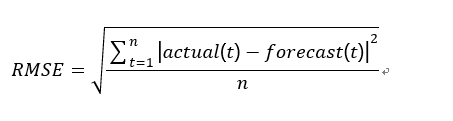import numpy as np

def mse_value(y_true, y_pred):
"""
参数:
y_true -- 测试集目标真实值
y_pred -- 测试集目标预测值

返回:
mse -- MSE 评价指标
"""

n = len(y_true)
mse = sum(np.square(y_true - y_pred))/n
return mse

平均绝对百分比误差 MAPE

MAPE  是 MAD 的变形，它是一个百分比值，因此比其他统计量更容易理解。例如，如果 MAPE 为 5，则表示预测结果较真实结果平均偏离 5 %。MAPE 的计算公式如下：MAPE is used to measure the relative errors between the average test value and the real value on the test set. MAPE is defined as: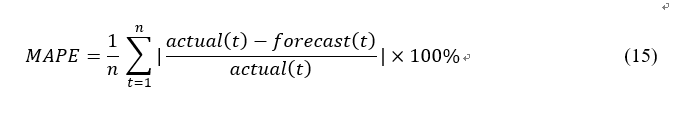MAPE 的值越小，说明预测模型拥有更好的精确度。使用 Python 实现 MSE 计算函数：

import numpy as np

def mape(y_true, y_pred):
"""
参数:
y_true -- 测试集目标真实值
y_pred -- 测试集目标预测值

返回:
mape -- MAPE 评价指标
"""

n = len(y_true)
mape = sum(np.abs((y_true - y_pred)/y_true))/n*100
return mape

展开全文• 我尝试在我的随机林代码中测量MAPE(平均绝对百分比误差)值。MAE值为7.5。当我试图计算MAPE时，它输出：Accuracy: -inf %这是我计算MAPE的代码。如何使它工作，或者为什么它不计算一个值。在^{pr2}$以下是值：errors:... 我尝试在我的随机林代码中测量MAPE(平均绝对百分比误差)值。MAE值为7.5。当我试图计算MAPE时，它输出：Accuracy: -inf % 这是我计算MAPE的代码。如何使它工作，或者为什么它不计算一个值。在 ^{pr2}$

以下是值：errors: array([ 2.165, 6.398, 2.814, ..., 21.268, 8.746, 11.63 ])

test_labels: array([45, 47, 98, ..., 87, 47, 72])

这些类型包括：var1 int64

var2 int64

var3 float64

var4 int64

var6 float64

var7 int64

var1. float64

dtype: object

示例值，超过8000个条目var1 var2. var3 var4 var5 var6 var7

"420823370" "183" "2019-09-07 22:13:04" "84" "2019-09-07 22:12:46" "72" "00:00:18"

"420521201" "183" "2019-09-07 17:43:03" "84" "2019-09-07 17:42:51" "46" "00:00:12"

"420219554" "183" "2019-09-07 12:43:02" "88" "2019-09-07 12:42:39" "72" "00:00:23"

"419618820" "183" "2019-09-07 02:43:01" "92" "2019-09-07 02:42:46" "80" "00:00:15"

"419618819" "183" "2019-09-07 02:43:01" "84" "2019-09-07 02:42:46" "80" "00:00:15"

"417193989" "183" "2019-09-05 10:42:52" "82" "2019-09-05 10:42:23" "0" "00:00:29"

"416891691" "183" "2019-09-05 05:42:51" "78" "2019-09-05 05:42:49" "72" "00:00:02"

"416587222" "183" "2019-09-05 00:42:51" "88" "2019-09-05 00:42:35" "99" "00:00:16"

"416587223" "183" "2019-09-05 00:42:51" "82" "2019-09-05 00:42:35" "99" "00:00:16"

"416587224" "183" "2019-09-05 00:42:51" "80" "2019-09-05 00:42:35" "99" "00:00:16"

id:Big Int. ts_tuid: Big Int. rssi: numeric. batl: real. ts_diff:interval

下面是代码示例：

从CSV加载数据model = (

pd.read_csv("source.csv", parse_dates=['var3', 'var5'], date_parser=lambda x: pd.to_datetime(x))

.assign(

rssi_ts=lambda x: x.loc[:, 'var3'].astype(int) / 10 ** 9,

batl_ts=lambda x: x.loc[:, 'var5'].astype(int) / 10 ** 9,

ts_diff=lambda x: pd.to_timedelta(x.loc[:, 'ts_diff']).astype(int) / 10 ** 9

)

)

# Labels are the values we want to predict

labels_b = np.array(halti['var4'])

# Remove the labels from the features

# axis 1 refers to the columns

features_r = halti.drop('var4', axis = 1)

features_r2 = list(features_r.columns)

# Convert to numpy array

features_r = np.array(features_r)

# Using Skicit-learn to split data into training and testing sets

from sklearn.model_selection import train_test_split

# Split the data into training and testing sets

train_features, test_features, train_labels, test_labels = train_test_split(features_r, labels_b, test_size = 0.25, random_state = 42)

# Import the model we are using

from sklearn.ensemble import RandomForestRegressor

# Instantiate model with 1000 decision trees

rf = RandomForestRegressor(n_estimators = 1000, random_state = 42)

# Train the model on training data

rf.fit(train_features, train_labels);

# Use the forest's predict method on the test data

predictions = rf.predict(test_features)

# Calculate the absolute errors

errors = abs(predictions - test_labels)

# Print out the mean absolute error (mae)

print('Mean Absolute Error:', round(np.mean(errors), 2), 'degrees.')

mape = 100 * (errors / test_labels)

# Calculate and display accuracy

accuracy = 100 - np.mean(mape)

print('Accuracy:', round(accuracy, 2), '%.')

展开全文java的mape
• 准确率：分类正确的样本占总样本个数的比例，Accuracy=ncorrect/ntotal 精确率Precision：精确率是指分类正确的正样本个数占分类器判定为正样本的样本个数...平均误差 RMSE（Root Mean Square Error）经常用来衡

准确率：分类正确的样本占总样本个数的比例，Accuracy=ncorrect/ntotal

精确率Precision：精确率是指分类正确的正样本个数占分类器判定为正样本的样本个数比例

召回率Recall：分类正确的正样本个数占真正的正样本个数的比例

如何评估排序模型的性能？

F1 Score和ROC曲线能综合反映一个排序模型的性能

F1=（2*Precision*Recall）/（Precision+Recall)

平均根误差

RMSE（Root Mean Square Error）经常用来衡量回归模型的好坏，但也有指标失效的时候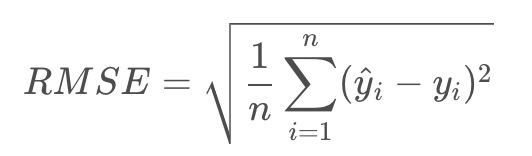其中：Yi是第i个样本点的真实值；^Yi是第i个样本点的预测值，m是样本点个数

一般情况下，RMSE能很好地反映回归模型预测值与真实偏离程度，但是在实际问题中，如果存在个别偏离程度非常大的离群点，即使离群点数量非常少，也会让RMSE指标变得很差

可以找个合适的指标来评估，比如MAPE，平均绝对百分比误差

MAPE：Mean Absolute Percentage Error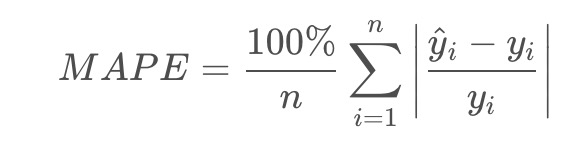展开全文机器学习
• 我们要编程计算所选直线的平均平方误差(MSE), 即数据集中每个点到直线的Y方向距离的平方的平均数，表达式如下： MSE=1n∑i=1n(yi−mxi−b)2 最初麻烦的写法 # TODO 实现以下函数并输出所选直线的MSE def ...
• matlab程序，用于MAE平均绝对误差，可直接在程序中以函数名的形式调用，用来检测模型的预测值与真实值之间的偏差，值越大表示预测效果越差
• import numpy as np from sklearn import metrics # 定义MAPE def mape(y_true, y_pred): return np.mean(np.abs((y_pred - y_true) / y_true)) * 100 # MSE print(metrics.mean_squared_error(y_true, y_pred)...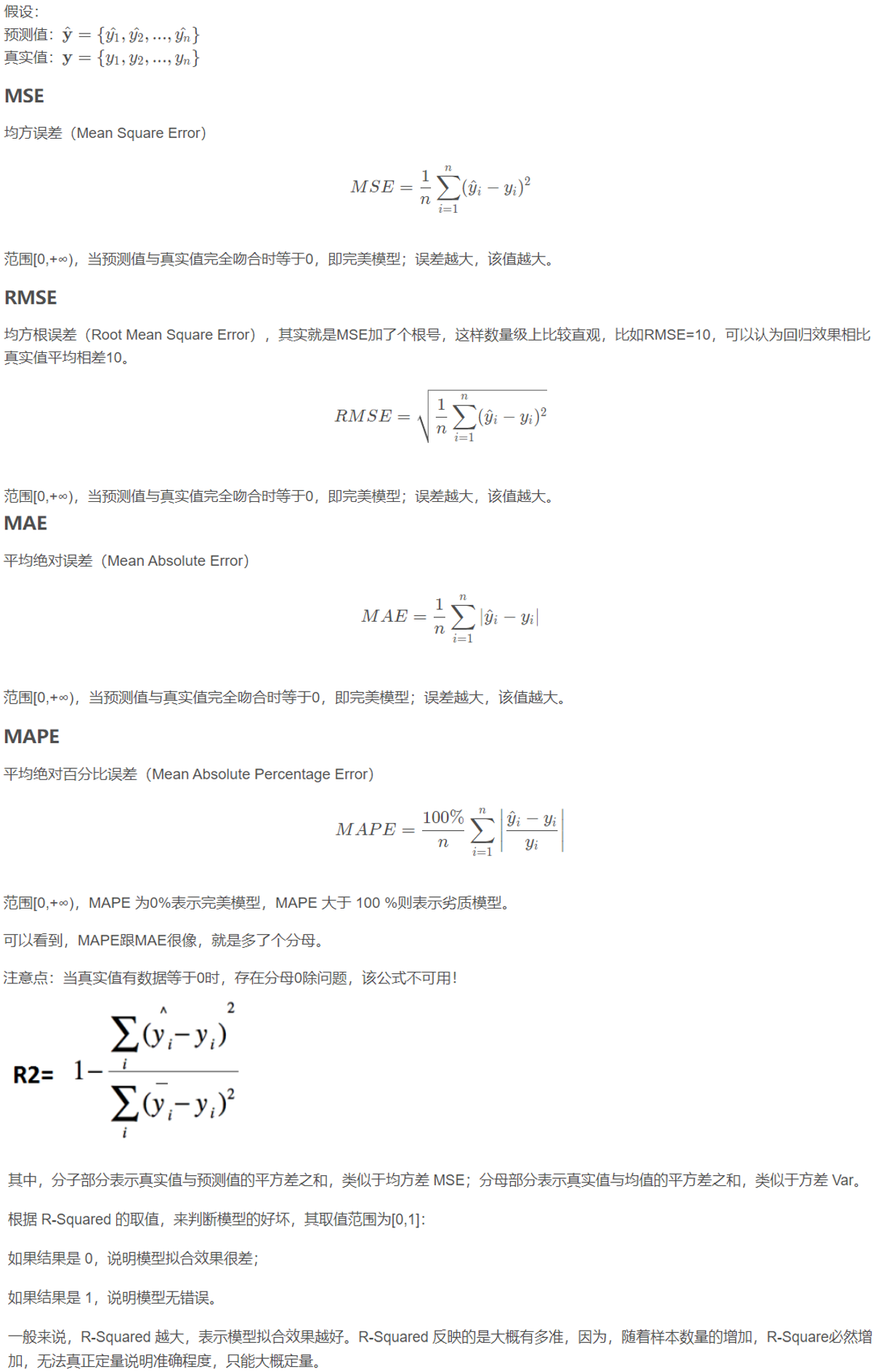代码

import numpy as np
from sklearn import metrics

# 定义MAPE
def mape(y_true, y_pred):
return np.mean(np.abs((y_pred - y_true) / y_true)) * 100%

# MSE
print(metrics.mean_squared_error(y_true, y_pred))
# RMSE
print(np.sqrt(metrics.mean_squared_error(y_true, y_pred)))
# MAE
print(metrics.mean_absolute_error(y_true, y_pred))
# R2
print(metrics.r2_score(y_ture,y_pred))
# MAPE
print(mape(y_true, y_pred))

展开全文python
• MAPE 平均绝对百分误差

万次阅读 2018-09-03 16:49:00
mape平均绝对百分误差 定义 def evalmape (preds, dtrain): gaps = dtrain.get_label() err = abs(gaps-preds)/gaps err[(gaps== 0)] = 0 err = np.mean(err) * 100 return 'error',err
• 模型评价：回归模型的常用评价指标1) ...模型越准确3) 较为好解释的评价指标：平均绝对误差(MAE)指标解释：所有样本的样本误差的绝对值的均值指标解读：平均绝对误差的单位与因变量单位一致，越接近0，模型越准确4...
• 计算你的折叠中的训练和测试错误，然后使用列表来附加结果。最后，绘制结果执行以下操作：from sklearn.neural_network import MLPRegressorfrom sklearn.metrics import mean_absolute_errorimport numpy as npfrom...
• 平均绝对偏差（MAE）： 计算公式： 优点：平均绝对误差由于离差被绝对值化，不会出现正负相抵消的情况，因而，平均绝对误差能更好地反映预测值误差的实际情况
• Mean Absolute Error ，平均绝对误差 是绝对误差的平均值 能更好地反映预测值误差的实际情况. 标准差 Standard Deviation ，标准差 是方差的算数平方根 是用来衡量一组数自身的离散程度 R...机器学习
• 1、标准差:标准差是方差的... --------------------------------------------------------------------------------------------------------2、均方误差：是指参数估计值与参数真值之差平方的期望值(英文解释-MSE: ...
• Mean Absolute Error ，平均绝对误差 是绝对误差的平均值 能更好地反映预测值误差的实际情况. 标准差 Standard Deviation ，标准差 是方差的算数平方根 是用来衡量一组数自身的离散程度...
• ", sum(absError) / len(absError))#平均绝对误差MAE aDeviation = [] aMean = sum(a) / len(a)#a平均值 for val in a: aDeviation.append((val - aMean) * (val - aMean)) print("a efficiency = ", 1-sum...
• 一、MeanAbsoluteError类 tf.keras.losses.MeanAbsoluteError( reduction=losses_utils.ReductionV2.AUTO, name=...均方误差平均绝对值误差只是计算方法不同，其他都是一样的。对应的是MeanSquaredError类和MSE函数。深度学习 tensorflow
• 这里有一个量化的NumPy的方法 -# From this post : http://stackoverflow.com/a/40085052/3293881def strided_app(a, L, S): # Window len = L, Stride len/stepsize = Snrows = ((a.size-L)//S)+1n = a.strides...
• 时间序列预测误差 时间序列-误差指标 (Time Series - Error Metrics) Advertisements 广告 Previous Page 上一页 Next Page 下一页 It is important for us to quantify the performance of a model ...机器学习 python 数据分析 深度学习 大数据
• 根据我从文档中读到的，它说平方误差的单位与响应的单位相同。有没有办法用百分比表示一个RMSE的值？例如，说这个百分比的预测是正确的，这是大错特错的。 在最新版本的sklearn中，有一个用于计算mean absolute ...
• 平均绝对百分比误差（MAPE） 平均绝对百分比误差（Mean Absolute Percentage Error，MAPE），平均绝对百分比误差之所以可以描述准确度是因为平均绝对百分比误差本身常用于衡量预测准确性的统计指标,如时间序列的...机器学习 人工智能 python 预测评价指标 MSE
• 平均绝对偏差、平均绝对误差 (MAE) 是所有单个观测值与算术平均值的偏差的绝对值的平均。与平均误差相比，平均绝对误差由于离差被绝对值化，不会出现正负相抵消的情况，因而，平均绝对误差能更好地反映预测值误差...
• Loading...## 残差平方和(SSE)### 计算公式：![sse](https://lpg-it.gitee.io/image/img/other/sse.png)### 代码实现：matlabsse...## 均方误差(MSE)### 计算公式：![mse](https://lpg-it.gitee.io/image/img/...
• 准确率 常用准确率 accuracy weighted accuracy MAE 平均绝对误差 ...MAPE 平均绝对误差百分比 适用 ： 对于补货计划来说，我们计算预测时间段 n代表n天。 SMAPE 权重 据对平方误差百分比 Yt是 t算法 机器学习 人工智能
• 2. 绝对轨迹误差（ATE） 直接计算相机位姿的真实值与SLAM系统的估计值之间的差值，首先将真实值与估计值的时间戳对齐，然后计算每对位姿之间的差值，适合于评估SLAM系统的性能。 参考链接：参考文章 ...自动驾驶 算法
• tensorflow 2.0 画出model网络模型的拓扑图 tensorflow 2.0 的回调函数callbacks（TensorBoard、ModelCheckpoint） ...MSE(均方误差)、MAE(平均绝对误差) from_logits CE(Cross-Entropy)、BCE(Binary Cross-Ent...
• MSE，RMSE，MAE，MAPE，sMAPE…等等有大量不同的错误度量标准，每个错误度量标准都有其...MAPE 平均绝对百分比误差（Mean Absolute Percentage Error） 平均绝对百分比误差是用于评估预测性能的最受欢迎的指标之一。 由机器学习 mse 度量指标
• 绝对轨迹误差 直接计算相机位姿的真实值与SLAM系统的估计值之间的差，程序首先根据位姿的时间戳将真实值和估计值进行对齐， 然后计算每对位姿之间的差值， 并最终以图表的形式输出， 该标准非常适合于评估视觉 SLAM...
• 1、绘制拟合曲线由前两章可知，时间序列分析模型为： Yt=0.9711×Yt−1−0.2664×ut−0.0793×ut−2+utY_t=0.9711\times Y_{...下面根据该模型来绘制拟合曲线，并计算拟合值与实际值的误差，代码如下：n (indata) u (0r语言...